# Fouling and its effect on the thermal performance of heat exchanger tubes

Fouling and its effect on the thermal performance of heat exchanger tubes

Department of Mechanical Engineering, College of Engineering, Andhra University, Visakhapatnam 530003, India

Department of Mechanical Engineering, K L University, Guntur 522502, India

Department of Mechanical Engineering, JNTUH College of Engineering, Hyderabad 500085, India

Department of Mechanical Engineering, GITAM University, Visakhapatnam 530045, India

Department of Mechanical Engineering, G.V.P. College of Engineering, Visakhapatnam 530048, India

Director, Indian Institute of Petroleum and Energy, Visakhapatnam 530003, India

Corresponding Author Email:
drkrk@kluniversity.in
Page:
509-519
|
DOI:
https://doi.org/10.18280/ijht.350307
|
Accepted:
|
Published:
30 September 2017
| Citation

OPEN ACCESS

Abstract:

The phenomena of fouling and corrosion in heat exchanger tubes commonly encountered in industrial practice are reviewed in two phases. In the first phase, the influence of physical and chemical properties of petroleum crude on scaling and fouling are reviewed. Further, in the second phase a generalized hypothesis is provided for unsteady analysis as function of time with the aid of logarithmic, exponential and Power law variation of fouling factors with time. The time dependent thermal characteristics of typical parallel and counter flow heat exchangers are established with the aid of time dependent fouling factors. The approach presented is in good agreement with the reported values of a petroleum refinery. Thus, a method to estimate the critical period for maintenance of the heat exchanger is established treating fouling as a time variable occurrence.

Keywords:

fouling, heat exchangers, maintenance, critical period, unsteady state

1. Introduction

Fouling of heat exchanger tubes is a complex phenomenon affecting the thermal performance of the heat exchange process which is commonly encountered in desalination, crude oil distillation plants, high power generation units and chemical industries. It reduces the heat transfer and decreases the overall thermal efficiency of the unit. Fouling in heat exchangers causes gradual impairment of its performance leading to increased pumping power. In addition, if timely cleaning is not scheduled it leads to severe penalty on operating costs . Taborek  identified the origin of the first industrial concern on fouling while Orrok  in 1910 was the first to mention fouling in the literature. Fouling has been treated as a major universal problem faced by the maintenance professionals of heat exchanger units  in industry. Hence, it is treated as a major concern in the design and operation of heat exchanger equipment . Fouling process involves transportation or diffusion of species from the medium towards the surface and the deposition increases progressively. This phenomenon will lead to fouling of either both hot and cold tubes or one of the two surfaces and rapidly increases the thermal resistance for heat transfer from the hot to the cold medium. The causes and effect of fouling in desalination and distillation of water and crude oil is detailed in the following sections.

1.1 Fouling with water

The flow of water influences both scaling and corrosiveness in heat exchanger tubes. The factors such as alkalinity, hardness, pH value, the quality of tube material, stray electrical currents and the presence of oxidizing agents like carbon dioxide, influence the scaling processes. The dissolved solids can further augment corrosion. In general, corrosion is the result of low pH of water. Acidic waters have a large number of H+ ions to react with the electrons at the cathode leading to corrosion. In contrast, water with a higher pH lowers the solubility of calcium carbonate so that the calcium carbonate is more likely to precipitate out as scale.  Hard water typically contains calcium compounds which can precipitate as calcium carbonate. However, if the hardness in the water is noncarbonated, the chlorate and sulfate ions will tend to keep the calcium in solution and will prevent scale formation. Other chemicals and compounds found in water also influence the corrosion process. The most common of these are oxygen, carbon dioxide, and dissolved solids. Oxygen reacts with hydrogen gas at the cathode, causing depolarization and speeding up corrosion. As a result, water with a high dissolved oxygen will tend to be corrosive. Other oxidizing agents can perform the same function, although they are less common. Nitrates and chlorine are two other oxidizing agents found in water.  Carbon dioxide in water also tends to cause corrosion. The carbon dioxide gas can combine with water to form carbonic acid, which lowers the pH of water. Dissolved solids are typically present in water as ions which increase the electrical conductivity of the water, making the electrolyte more effective resulting in increased corrosion rate. Alkalinity is a measure of how easily the pH value of water can change and hence can be considered to have a mitigating influence with pH. Water with a high alkalinity is more likely to form scales. In contrast, low alkalinity water lacks the buffering capacity to deal with acids, so they can easily become acidic and corrosive.

The relationship between pH, alkalinity, and water stability is depicted by the Baylis graph as shown in figure 1. Water above the lines is scale-forming while water below the lines is corrosive.  Stable water is found in the white area between the lines.

## 1.pngFigure 1. Baylis Graph (variation of pH with Alkalinity)

The physical characteristics of liquids viz., temperature and velocity of flow can influence corrosion. The rate of corrosion increases with temperature which is a complex phenomenon. A high temperature reduces the solubility of calcium carbonate in water, which promotes scale formation and decelerates corrosion. Temperature also alters the form of corrosion.  Pits and tubercles tend to form in cold water while hot water promotes uniform corrosion. Uniform corrosion spreading across the entire surface of a pipe is far less problematic than tuberculation, so high temperatures can actually seem to slow the corrosive process. The variation of pH with water temperature is given by the relationship as:

$p H=7.465-0.0203 T+7.065 \times 10^{-5} T^{2}$     (1)

The influence of flow velocity on corrosion is also rather complex. Moderate flow rates are beneficial since they promote the formation of scale without breaking loose tubercles. At low flow velocities, corrosion rate increase and tend to be in the form of tuberculation. At very high flow velocities, abrasion of water against the pipe tends to a different form of corrosion. At high flow velocities, the protective scale and tubercles is removed which increase the contact of the pipe with oxygen and lead to increase the rate of corrosion. A more uniform and homogeneous distribution of the velocity results in less formation of fouling and, consequently, fewer erosions and corrosions inside the shell.

1.2 Fouling with crude oil

Crude oil, one of the most significant fossil fuels, is basically a mixture of different hydrocarbons. It is categorized based on the proportion of asphaltenes and Sulphur as light, medium and heavy oil. Characterization of crude oils were performed by Young et al. , with a batch stirred cell system to study crude oil fouling for surface temperatures up to 4000C and pressures up to 30 bars. The primary task involved in the processing of crude oil is distillation which is based on volatility. In the distillation of crude oil, the disassociated components are hot and play a prominent role in transferring the heat in a series of heat exchangers known as Pre-Heat Train (PHT) against the incoming crude oil. The heavy compounds in the crude tend to disassociate through physical or chemical processes with deposits observed on the heat exchanger walls . This phenomenon known as ‘fouling’ affects the thermodynamic and hydrodynamic efficiency of heat exchangers . Hazmi et al.  estimated the impact of fouling on heat transfer performance of a plate heat exchanger and found  its overall hetatransfer coefficient drops by  50% .

Fouling of crude-oil is due to hydrocarbon oxidation or auto-oxidation, asphaltenes precipitation and wax deposition. This will not only decrease the performance of heat exchangers but also obstruct the flow creating a blockage. The hydrocarbon oxidation takes place when the temperature and crude-containing oxygen concentrations reach a certain value of activation energy. The asphaltenes precipitation is activated by temperature, pressure and concentration fluctuation of the crude . When the oil cools, the aggregation and deposition of wax takes place on the walls of the tube or surface. If the fouling is reaction controlled, the rate of fouling decreases with flow velocity  where as if the fouling rate is mass transfer controlled, the fouling rate increases with flow velocity.

The fouling mechanisms are observed to follow a pattern starting with initiation or delay period, transportation, deposition, removal and aging. The important factors influencing fouling are: Surface temperature, Bulk temperature, Type of Crude, Crude Blending and Flow velocity.

An increase in surface temperature increases the fouling rate exponentially which is observed in all mechanisms [13-17]. However, the effect of bulk temperature has contradicting observations with Lambourn and Durrieu , Fuhr et al., , Storm et al. . They reported an increase in fouling with decrease in bulk temperature. Several studies on the deposition from petroleum feed stocks were reviewed and observed that the deposition is due to particulates and gums for low Sulphur crude oils whereas it is due to formation of iron sulphides for medium Sulphur crude oils. Higher proportion of asphaltenes and Sulphur observed in heavy oils tend to foul faster compared to medium and light oils . Crude oils from different sources when blended can result in rapid fouling  and coking in PHT. In order to predict the proportion of blending oils which would avoid incompatibility, certain crude oil tests were developed .

The rate of fouling may increase or decrease with the increase of velocity in PHT depending on approach to fouling control. The influence of flow velocity, bulk temperature, surface temperature and particulate concentrations are demonstrated through experimental results, and compared with numerical results by Watkinson .

Jamialahmadi et al.  conducted experiments to determine the rate of deposition of flocculated asphaltenes particles in oil and compared with theoretical results under forced convective conditions using thermal approach. The effect of oil velocity, flocculated asphaltenes concentration and temperature on the rate of asphaltenes deposition is investigated. It has been reported that in the early weeks, the deposition mechanism is quite significant whereas the erosion of the deposit is quite ineffective. The predictions of the model for the deposition rate of asphaltenes are compared with measured data and the results are observed to be in good agreement.

1.2.1 Bacteria

Bacteria can cause and also accelerate the rate of corrosion. In general, bacterial colonies on pipe walls accelerate corrosion due to oxygen cell concentration below them, causing increased pitting and tuberculation. Like humans, some bacteria produce carbon dioxide, which can combine with water to become carbonic acid and accelerate corrosion. The bacterial colonies also block the deposition of calcium carbonate scale on the pipe walls.

There are two main types of corrosion-related bacteria, each of which causes its own set of additional corrosion problems. Iron bacteria use the ferrous iron created at the anode, converting it into rust which they deposit in the slime around their cells.  Since they use up the ferrous iron, this increases the rate of corrosion. Their slime can also come loose during high flow velocities, causing red water complaints and a bad smell. Sulfate-reducing bacteria use up sulfate in the water to produce hydrogen sulfide. Hydrogen sulfide is an acid which can react with metals, causing corrosion. In addition, the sulfides produce a distinctive rotten egg smell. The effect of bacteria on ferrous Iron is shown in figure 2 .

## 2.pngFigure 2. A colony of iron bacteria 

To avoid fouling of the tubes either due to scaling or corrosion, the preferred pH value of the water is to be ensured in the limits of -5% to +5%.

1.2.2 Other factors

Other than water properties, and bacteria the metal pipe characteristics and electrical currents are common causes of corrosion. Metals higher on the galvanic series tend to be more corrosive while metals farther apart on the series are more likely to cause galvanic corrosion. In galvanic corrosion, the size of the cathode in relation to the anode has a large influence on corrosion as well. Larger cathodes tend to promote corrosion by speeding the electrical current's flow. When a system has very small anodes and large cathodes, corrosion is so rapid at the anodes that pinholes tend to form all the way through the metal. Stray electrical current can cause electrolytic corrosion. Electrolysis usually causes problems on the outsides of pipes.

1.3 Temperature dependent of the pH of pure water

Another important variable is temperature in a heat exchanger which influences all the scale forming and corrosive characteristics. The formation of hydrogen ions (hydroxonium ions) and hydroxide ions from water is an endothermic process. The simpler version of the equilibrium equation is

$H_{2} O \Leftrightarrow H+(a q)+O H-(a q)$       (2)

Hence, the forward reaction, as written, absorbs heat. According to Le Châtelier's Principle, any changes made to the conditions of a reaction in dynamic equilibrium will lead to a change in the position of equilibrium. Hence, if there is an increase in the temperature of the water, the equilibrium will move to lower the temperature again. It will do that by absorbing the extra heat. That means the forward reaction will be favored, and more hydrogen ions and hydroxide ions will be formed. The effect of that is to increase the value of Ion product of water (Kw) as temperature increases. The Table.1 below shows the effect of temperature on Kw. For each value of Kw, a new pH has been calculated.

Table 1. Effect of temperature on pH value of water

 T (°C) Kw (mol2 dm-6) pH 0 0.114 x 10-14 7.47 10 0.293 x 10-14 7.27 20 0.681 x 10-14 7.08 25 1.008 x 10-14 7.00 30 1.471 x 10-14 6.92 40 2.916 x 10-14 6.77 50 5.476 x 10-14 6.63 100 51.3 x 10-14 6.14

## 3.pngFigure 3. Variation of pH of water with temperature

The variation of pH with respect to temperature will be given by the relationship as follows:

$p H=7.465-0.0203 T+7.065 \times 10^{-5} T^{2}$     (3)

It is observed from the above equation and figure 3 that the pH of pure water decreases as the temperature increases. It does not mean that water becomes more acidic at higher temperatures. A solution is acidic if there is an excess of hydrogen ions over hydroxide ions (i.e., pH < pOH). In the case of pure water, there are always the same concentration of hydrogen ions and hydroxide ions and hence, the water is still neutral (pH = pOH) - even if its pH changes.

The problem is that with 7 being the pH of pure water, that anything else is felt really strange. Hence calculate the neutral value of pH from Kw. If that changes, then the neutral value for pH changes as well. At 100°C, the pH of pure water is 6.14, which is "neutral" on the pH scale at this higher temperature. A solution with a pH of 7 at this temperature is slightly alkaline because its pH is a bit higher than the neutral value of 6.14.

Similarly, a solution with a pH of 7 at 0°C is slightly acidic, because its pH is a bit lower than the neutral value of 7.47 at this temperature.

1.4 Scope of the present investigation

In industrial heat exchangers, the coolant ponds conserve the coolant and the bacterial contamination will be inevitably present due to constant exposure to the ambient surroundings. This in turn will affect the pH value of the coolant and its alkalinity.  This will promote the undesirable influence of the coolant circulated in heat exchanger tubes. In course of time the heat exchanger tubes must be treated for elimination of both corrosive and scaling effects for better thermal performance. Besides, fouling can occur due to scaling of certain components like asphaltenes in the base fluid like in petroleum crude on the surface separating hot and cold fluids.

The specific objectives of the present work are:

► To propose a hypothesis to estimate fouling through a typical case of impairment of thermal performance of flow heat exchanger

► To determine the thermal resistance at the tube wall. i.e. the fouling thickness on the tube wall with respect to time by applying principles of analogy between heat and mass transfer.

► To estimation of the % enhanced power requirement for changing conditions of live flow area in the tube due to fouling.

The crude from different geographic sources going to refinery might be different in several aspects with regard to its constituents and chemical compositions. Hence the mass diffusivity might be different. To reflect this aspect in the analysis different approaches of fouling rates such as logarithmic, exponential, power law functions are considered. In conclusion, the results of petroleum refinery at Tehran are taken from the literature for comparison. The orders of magnitude of predictions from the present analysis are in close proximity of the results of Tehran petroleum industry.

2. Formulation

The formulation is further accomplished with the following assumptions. The time dependent nature of the exit temperature of the hot fluid is assumed to take place because of the fouling resistance at the periphery of the hot tube. It is further assumed the heat flux at the wall of the hot tube is uniformly same all along the inner wall of the hot tube but time dependent. The thermal resistance is dependent on the degree of fouling. The analysis pertains to the simplest cases of either parallel or counter flow heat exchanger.

The thermo-physical properties of both hot and cold fluids are independent of thermal changes. The overall heat transfer coefficient changes with time since the fouling factor is time dependent.  Besides, the principles of analogy between heat and mass transfer are equally applicable to transport phenomena in heat exchangers. The flow rates of both hot and cold fluids are constant and time invariant.

The physical and thermal properties of the petroleum products are computed from the following relationships .

Table 2. Property relations for crude oil

 $\rho_{0}=834.4-0.276 T_{b}$ $\mu_{o}=\left[0.01927-\left(1.3 e^{-4}\right) T_{b}+\left(2.2085 e^{-7}\right) T_{b}^{2}\right]$ $E x p\left[568.84-1.51\left(T_{b} / 33.28\right)\right]$ $k_{o}=0.145-0.0001 T_{b}$ $C_{o}=1671+4.45 T_{b}$

Fouling is an independent variable mostly influenced by the quality of locally available cooling water and petroleum product. Fouling can be represented either by logarithmic, exponential or power functions of heat flux at the inner periphery solely as functions of period of operations typically number of days. Thus, the following functions are considered in the hypothesis.

Table 3. Property relations for water are as follows:

 $\rho_{H_{2} O}=1000 \times$ $\left[1.0-\frac{(T-4.0)^{2}}{119000+1365 \times T-4 \times T^{2}}\right]$ $\mu_{H_{2} O}=0.00169-4.25263 e^{-5} \times T+4.9255 e^{-7}$ $\times(T)^{2}-2.0993504 e^{-9} \times(T)^{3}$ $k_{H_{2} O}=0.56112+0.00193 \times T-2.6015274 e^{-6}$ $\times(T)^{2}-6.08803 e^{-8} \times(T)^{3}$ $C_{H_{2} O}=4217 \cdot 629-3.20888 \times T+0.09503 \times$ $(T)^{2}-0.00132 \times(T)^{3}+9.415 e^{-6} \times$ $(T)^{4}-2.5479 e^{-8} \times(T)^{5}$

Logarithmic function

$\frac{Q(\tau)}{Q(\tau=0)}=R^{+}(\tau)=\left[\frac{1}{B \log (1+B \tau)}\right]$         (4)

$\frac{Q(\tau)}{Q(\tau=0)}=R^{+}(\tau)=\left[\frac{1}{1+B \log (1+\tau)}\right]$    (5)

Exponential function

$\frac{Q(\tau)}{Q(\tau=0)}=R^{+}(\tau)=\operatorname{Exp}(-B \tau)$      (6)

Power law

$\frac{Q(\tau)}{Q(\tau=0)}=R^{+}(\tau)=1-B\left[\frac{\tau}{\tau^{*}}\right]^{1 / 7}$       (7)

$\frac{Q(\tau)}{Q(\tau=0)}=R^{+}(\tau)=1-B\left[\frac{\tau}{\tau^{*}}\right]^{0.1}$       (8)

B is a variable constant dependent on the fouling rates to be dependent on system variables and the quality of the hot and cold fluids  is critical time.

## 4.png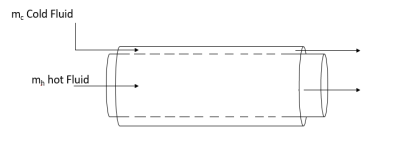Figure 4. Configuration of parallel flow concentric tube heat exchanger

The performance of a tube in a tube heat exchanger can be formulated for the configuration shown in figure 4. From the foregoing study, it is evident that fouling of the tubes for several reasons will impair the heat exchange between hot and cold fluids and the effectiveness will be decreasing with time. Hence the heat exchanger tubes are to be cleaned and the fouling is to be removed. Thus, the subsequent formulation is presented in the estimation of hot and cold fluid temperatures with respect to time for an elemental length dz shown in figure 5.

## 5.pngFigure 5. Hot fluid element

The conservation of energy for the control volume of the hot fluid element is as follows:

$Q_{h 1}=m_{h} C_{h} T_{h}$      (9)

$Q_{h 2}=m_{h} C_{h} T_{h}+m_{h} C_{h} \frac{\partial T_{h}}{\partial Z} d Z$      (10)

$Q_{w}=q_{w} \pi D d Z$     (11)

$Q_{\tau}=\rho_{h} A_{h} C_{h} \frac{\partial T_{h}}{\partial \tau} d Z$      (12)

$Q_{h 1}=Q_{h 2}+Q_{w}+Q_{\tau}$      (13)

$\frac{\partial T_{h}}{\partial Z}+\frac{q_{w} \pi D}{m_{h} C_{h}}+\lambda \frac{\partial T_{h}}{\partial \tau}=0$        (14)

where

$\lambda=\left(\pi D^{2} \rho_{h}\right) / 4 m_{h}$     (15)

$T^{+}=\frac{T_{h i}-T_{h}(\tau)}{T_{h i}-T_{h}(\tau=0)}$      (16)

Thus, the unsteady nature of the phenomenon is because of time varying nature of the fouling factor at the surface of separation between hot and cold fluids. It is further assumed that the heat flux at this plane is purely a function of time to be defined by a functional relationship.

$q_{w}=q_{w}(\tau)$       (17)

Therefore, said discussion on fouling is suggestive that it is a progressive time varying phenomenon affecting the effectiveness of heat exchange between the hot and cold fluids. Eq. (14) can be converted into dimensionless form as follows

$\left[\frac{\partial T^{+}}{\partial \eta}\right]-\frac{Q\left(\tau^{+}\right)}{Q\left(\tau^{+}=0\right)}-\left(\frac{\lambda}{4 \tau^{+}}\right)\left[\frac{\partial T^{+}}{\partial \tau^{+}}\right]=0$          (18)

Employing integral method Eq. (18) can be written as follows:

$\int_{\eta=0}^{\eta=1} \partial T^{+}-\frac{Q\left(\tau^{+}\right)}{Q\left(\tau^{+}=0\right)} \int_{0}^{1} d \eta-\lambda \frac{d}{d \tau^{+}}\left[\int_{\eta=0}^{\eta=1} T^{+} d \eta\right]=0$       (19)

The solution for equation is assumed as

$T^{+}=\eta^{3} F\left(\tau^{+}\right)$      (20)

Substituting Eq. (20) in Eq. (19) will yield differential Eq. (21) for thermal conditions at.

$d F\left(\tau^{+}\right)+\lambda^{*}\left[F\left(\tau^{+}\right)-R\left(\tau^{+}\right)\right]=0$      (21)

where $\lambda^{*}=\frac{\lambda}{4} d \tau^{+}$

The boundary conditions at are as follows:

$F\left[\tau^{+}=0\right]=1$ and $F\left[\tau^{+}\right]=F_{c}$                                (22)

With the objective to determine $\lambda^{*}=\lambda_{c}^{*}$ when $\tau^{+}=1$ . From $\lambda_{c}^{*}$ , the number of days elapsed for fouling to impair thermal transport to cold fluid can be evaluated.

Variation of fouling thickness with time

The fouling at the periphery can be evaluated from the differential equation, given below

$\frac{1}{4} \frac{d}{d \tau}\left[\pi d^{2}-\pi(d-2 \delta)^{2}\right]=h_{m} \pi(d-2 \delta)$       (23)

$\left[\frac{d \delta}{d \tau}\right]=h_{m}$      (24)

where hm related to the specific species in the base fluid diffusing towards the periphery of the tube and d is fouling thickness a time variable to be assessed. Assuming that the principle of analogy between heat and mass transfer is valid, Eq. (24) can be written as

$\left[\frac{d \delta^{+}}{d \tau^{+}}\right]=\left[\frac{h_{m} \tau^{*}}{d}\right]$       (25)

In the present study, the mass diffusivity of the component (asphaltenes) in the petroleum base towards the wall is assumed as 1e-10 m/s.

Thus,

$\left[\frac{d \delta^{+}}{d \tau^{+}}\right]=N u\left[\frac{\alpha_{m} \tau^{*}}{d^{2}}\right]$ where $N u=\left[\frac{h_{\text {con}} d}{k}\right]$        (26)

where

$\delta^{+}=\frac{\delta}{d} ; \tau^{+}=\frac{\tau}{\tau^{*}}$        (27)

2.2 Void ratio

Further, the void ratio for the case of fouling is defined as

Void Ratio$=\left[\frac{A_{f}}{A}\right]=\left[4 \delta^{+}-\delta^{+2}\right]$       (28)

where Af and A are respectively the cross-sectional areas with and without fouling.

$A^{+}=\left(1-\frac{A_{f}}{A}\right) \times 100$       (29)

where A+  is % Change in flow area

2.3 Pressure gradient ratio ($\beta$)

The pressure gradient associated with the flow for non-fouling to the fouling case can be computed from the relationship

$\left[\frac{\Delta P}{L}\right]_{\text { non-foulling }}=\left[\frac{C}{d^{1.8}}\right]$     (30a)

$\left[\frac{\Delta P}{L}\right]_{\text { fouling }}=\left[\frac{C}{[d-2 \delta]^{1.8}}\right]$          (30b)

where $\quad C=\left[\frac{0.023 \times 4^{2.8} \mu^{0.2} m^{1.8}}{\pi^{1.8} \rho}\right]$

Eqs. (30a, 30b) are derived for turbulent flow conditions of the base fluid at constant flow rate for both fouling and non-fouling cases. The magnitude of the friction coefficient, f is computed from

$f=\frac{0.046}{\mathrm{Re}^{02}}$      (31)

Hence the pressure gradient ratio b is evaluated as

$\beta=100$[ $\left[\frac{\Delta P}{L}\right]_{\text {fouling}}-\left[\frac{\Delta P}{L}\right]_{\text {non-fouling}}$ / $\left[\frac{\Delta P}{L}\right]_{\text {non-fouling}}$ ]       (32)

2.4 Power Enhancement ratio ($\phi$) due to fouling

Power requirement for unit length of the tube can be computed from

$p=\left[\frac{\Delta P}{L}\right]\left[\frac{m}{\rho}\right]$    (33)

The power enhancement ratio $\phi$ can be computed from

$\varphi=100\left[\frac{1-\left(1-\frac{2 \delta}{d}\right)^{3.8}}{\left(1-\frac{2 \delta}{d}\right)^{3.8}}\right]_{\%}$     (34)

3. Solution Procedure

To obtain a general solution of the formulation made in the earlier section a petroleum product considered to be cooled from an initial temperature of 90℃ by water at an inlet temperature of 30℃. The length of the hot tube is taken as 4m with an outside diameter of 2.54 cm and inner diameter of 2.0 cm. If the fouling is inevitable due to change in the physical, chemical and bacterial properties of the coolant, then the above formulation is applied to estimate the change in the outlet temperature of the hot fluid with time. This is also used to estimate the days for maintenance of the tubes as per the afore presented hypothesis at $T^{*} \rightarrow 0.4$ . The nature of fouling is assumed to be dependent on time of operation by logarithmic, exponential and 1/7th power law (i.e., equations 5, 6 and 8). The parameters listed in equations 21 to 34 are calculated and presented by generating a FORTRAN program.

4. Results and Discussion

The formulations are further represented by the results shown in figures 6-18. The nature of variation of the fouling is typically represented by the various functional relationships shown in the figure 6. The logarithmic and 1/7th power law variations of fouling reveal very steep gradients with respect to time and the exponential function seems to be more realistic and desirable since the wall heat flux is affected gradually at the wall of the tube. Nevertheless, fouling is dependent on the external physical chemical and other variables of the hot and cold fluids as discussed earlier. The variable B in these equations is dependent on the external and other physical and chemical factors leading to fouling and it is an unknown dependent on the specific nature of hot and cold fluids. As a result of fouling the transport of heat from the hot fluid gets diminished gradually resulting in the rise of its exit temperature with respect to time. Figure 7 represents the gradual rise of the exit temperature of the hot fluid thus decreasing the effectiveness of heat exchange process

from hot fluid to the cold medium. The relative variations of R+ and T+ with respect to time of operation for the three types of fouling cases are depicted in figure 8.

Figures 9 to 14 are drawn to a specific case of a heat exchanger with assigned data of inlet temperatures for both hot and cold fluids with finite discharge rates. Figure 9 shows the nature of variation of the hot fluid exit temperature for the three types of fouling for a chosen inlet hot fluid temperature of 90℃. It reveals the drop-in heat exchange as the exit temperature of hot fluid rises with time of operation. For logarithmic fouling, the rise occurs at a faster rate compared to the other two cases. Figure 10 correspondingly shows that the heat of abstraction from the hot fluid by the cold medium is more in the case of logarithmic fouling than the other types of variations.

A typical variation of LMTD (logarithmic mean temperature differences) for exponential and 1/7th laws of fouling is shown in figures 11 and 12 respectively. It is observed that LMTD for counter flow system is higher than that of the case of parallel flow system and with time of operation the variation is becoming less.

The figures 13 and 14 shows variation of thermal resistance with respect to time of operation for parallel flow and counter flow respectively. The thermal resistance is found increasing with increase in time of operation. The thermal resistance for the exponential and 1/7th power law models coincides after long hour of operation as evident from the graphs.

Figure 15 shows the fouling behavior for the crude oil pre-heater at a petroleum refinery Tehran. The orders of magnitude of predictions obtained from the present analysis as shown in figures 13 and 14 are in close proximity of the results of Tehran petroleum industry .

The variation of thickness of the fouling d in mm with respect to time is shown in figure 16. It is clear that the fouling varies monotonically and the external factors leading to logarithmic fouling yields minimum thickness in relation to the other three shown in the plot. The variation of corresponding available flow area is indicated in figure 17. Figure 18 shows % enhancement of power due to fouling to maintain constant flow rate of the base fluid. It is observed from the figure that the % enhancement of  power  is  high  in

1/7th power law model. It is evident that the pressure rise is abnormally high to maintain constant flow rate over a reasonable period of operation for all types of fouling. Thus, power requirement to maintain constant flow rate increases with the period of operation. Maintenance of the heat exchangers is primarily dependent on the nature of external parameters of the cold and hot media in contact with the surface leading to the type of fouling. It is essential to prefix the nature of fouling for scheduling maintenance to avoid abnormally high-power ratings.

## 6.png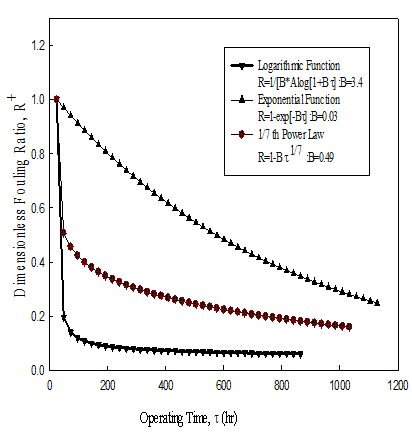Figure 6. Variation of dimensionless fouling ratio with operating time

## 7.png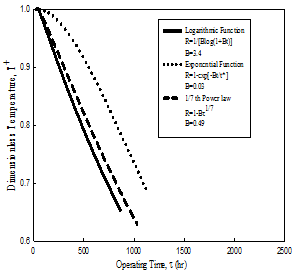Figure 7. Variation of dimensionless temperature with operating time

## 8.pngFigure 8. Comparative variations of dimensionless fouling ratio and dimensionless temperature with hours of operation

## 9.pngFigure 9. Variation of hot fluid temperature at inlet and exit for different fouling factors

## 10.png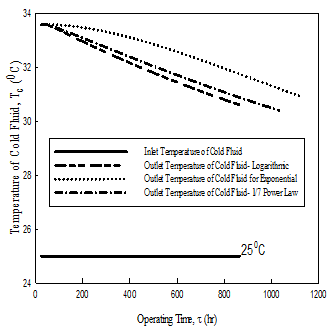Figure 10. Variation of cold fluid temperature for different fouling factors

## 11.pngFigure 11. Variation of LMTD with time of operation

## 12.png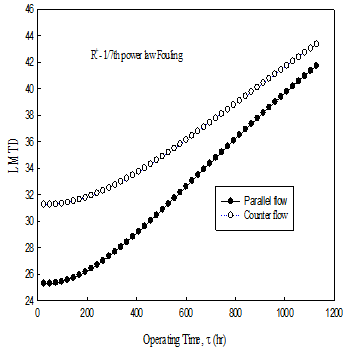Figure 12. Variation of LMTD with time of operation

## 13.png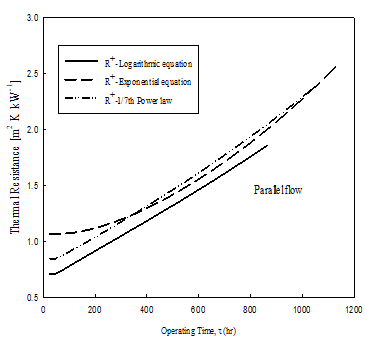Figure 13. Variation of thermal resistance with time of operation

## 14.pngFigure 14. Variation of thermal resistance with time of operation

## 15.png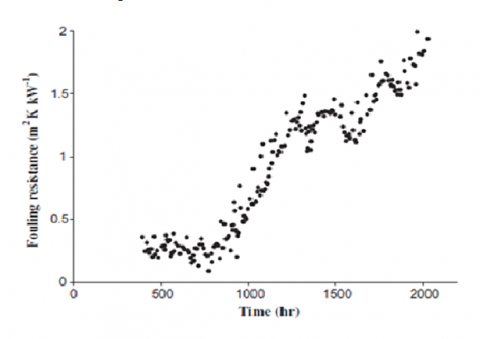Figure 15. Fouling resistance variation with respect to time data from petroleum refinery at Tehran 

## 16.pngFigure 16. Variation of fouling thickness with time of operation

## 17.pngFigure 17. Percentage change in flow area with time of operation

## 18.pngFigure 18. Percentage enhancement of power requirement due to fouling

5. Conclusions

The characteristics of fluid flow in tubes associated with fouling have been determined. The model predicts the duration of operation of heat exchanger under parallel flow and counter flow. The overall heat transfer coefficient decreases for both parallel and counter flow heat exchangers. The variation of fouling thickness with respect to time has been determined and compared with trend available in the literature for the assumed resistance profiles. The reduction in flow area of the tube considered up to 40% resulting in increase in power requirement is shown for different fouling ratios.

Thus, the results in various plots correspond to the following fouling factors:

Logarithmic function

$R^{+}(\tau)=\left[\frac{1}{B \log (1+B \tau)}\right]$       (35)

where $B=4.05\left\{\tau^{*}\right\}^{-0.1717}$

Average deviation = 1.45%

Standard deviation = 1.737%

Exponential function

$R^{+}(\tau)=1-E x p\left[-B \tau / \tau^{*}\right]$        (36)

where $B=0.1079\left(\tau^{*}\right)^{-0.0126}$

Average deviation = 0.189%

Standard deviation = 0.237%

Power law

$R^{+}(\tau)=\left[1-B \tau^{1 / 7}\right]$      (37)

where $B=0.905\left(\tau^{*}\right)^{-0.1438}$

Average deviation = 0.027%

Standard deviation = 0.034%

The fouling factors are corresponding to the following range of operation of the heat exchangers.

30 < τ Days < 500.

Out of the above three models presented the exponential variation of fouling rates seems to be in close agreement with the results of petroleum industry. However, the other predictions don’t deviate substantially as indicated in the figures 9 to 18. Hence the choice of selection of suitable model is left to the specific crude employed in the refinery.

Nomenclature
 A Area (m2) B Fouling Constant C Specific heat (J. kg-1. K-1) D Outer Diameter (m) d Inner Diameter (m) f Friction factor hm mass transfer Coefficient (m. s-1) k Thermal conductivity (W. m-1 K-1) Kw Ion Product of water (mol2. dm-6) L Length of the tube (m) LMTD Logarithmic Mean Temperature Difference m Mass Flow rate (kg. s-1) Nu Nusselt Number P Pressure (kPa) p Power (kW) pH Potential of Hydrogen Ion Q Heat transfer (kW) q Heat flux (kW. m-2) R Fouling Ratio Re Reynolds Number T Temperature (K) z Local position measured from entry to the hot tube Greek Symbols am Mass diffusivity (m2. s-1) b Pressure gradient ratio d Fouling Thickness (mm) h dimensionless distance (z. L-1) m Viscosity (kg. m-1 s-1) f Power Enhancement Ratio r Density (kg. m-3) τ Time(hrs.) Superscripts and Subscripts b bulk mean c Cold h Hot i inlet o oil w wall * critical + Non-dimensional
References

 Coletti F., Joshi H.M., Macchietto S., Hewitt G.F. (2015). Introduction, Crude Oil Fouling, Deposit Characterization, Measurements, and Modeling, F. Coletti and G.F. Hewitt (Eds.), Gulf Professional Publishing (Elsevier), Boston, USA. pp. 1-22. DOI: 10.1016/B978-0-12-801256-7.00001-4

 Taborek J. (1997). Assessment of fouling research on the design of heat exchangers, Fouling mitigation of industrial heat exchange equipment, Begell House Inc, New York.

 Orrok G. (1910). The transmission of heat in surface condensation, Trans. ASME, Vol. 32, pp. 1139-1214.

 Taborek J., Aoki T., Ritter R.B., Palen J.W., Knudsen J.G. (1972). Fouling: The major unresolved problem in heat transfer, Chemical Engineering Progress, Vol. 68, No. 2, pp. 59-67.

 Watkinson A.P., Panchal C. (1990). A critical review of organic fluid fouling, Argonne National Lab. IL (USA).

 Cucumo M., Ferraro V., Kaliakatsos D., Mele M., Galloro A., Schimio R., Le Pera G. (2016). Thermohydraulic analysis of a shell-and-tube “helical baffles” heat exchanger, International Journal of Heat and Technology, Vol. 34, No. Sp. 2, pp. S255-S262. DOI: 10.18280/ijht.34S210

 Young A., Venditti S., Berrueco C., Yang M., Waters A., Davies H., Hill S., Millan M., Crittenden B. (2011). Characterization of crude oils and their fouling deposits using a batch stirred cell system, Heat Transfer Engineering, Vol. 32, No. 3-4, pp. 216-227. DOI: 10.1080/01457632.2010.495603

 Epstein N. (1983). Thinking about heat transfer fouling: A 5× 5 matrix, Heat Transfer Engineering, Vol. 4, No.1, pp. 43-56.

 Yang J., Serratos M.G.J., Fari-Arole D.S., Müller E.A., Matar O.K. (2015). Crude oil fouling: Fluid dynamics, reactions and phase change, Procedia IUTAM, Vol. 15, pp. 186-193. DOI: 10.1016/j.piutam.2015.04.026

 Ahmad Syafiq Ahmad Hazmi, Zulina Abdul Maurad, Nik Nurfatmah Pz Nik Pauzi, Zailan Abu Bakar and Zainab Idris. (2016). Rapid evaluation of plate heat exchanger performance and fouling analysis in epoxidation of oleochemical at pilot plant scale, International Journal of Heat and Technology, Vol. 34, No. 4, pp. 558-564. DOI: 10.18280/ijht.340402

 Watkinson A., Wilson D. (1997). Chemical reaction fouling: A review, Experimental Thermal and Fluid Science, Vol. 14, No. 4, pp. 361-374.

 Asomaning S. (1997). Heat exchanger fouling by petroleum asphaltenes, Ph.D. dissertation, Department of Chemical and Bio-Resource Engineering, The University of British Columbia, Vancouver, Canada.

 Scarborough C., Cherrington D., Diener R., Golan L. (1979). Coking of crude oil at high heat flux levels, Chem. Eng. Prog, Vol. 75, No. 7, pp. 41-47.

 Eaton P., Lux R. (1984). Laboratory fouling test apparatus for hydrocarbon feedstocks, ASME-HTD, Vol. 35, No. 1, pp. 33-42.

 Crittenden B., Kolaczkowski S., Downey I. (1992). Fouling of crude oil preheat exchangers, Chemical Engineering Research & Design, Vol. 70, No. 6, pp. 547-557.

 Saleh Z.S., Sheikholeslami R., Watkinson A. (2005). Fouling characteristics of a light Australian crude oil, Heat Transfer Engineering, Vol. 26, No. 1, pp. 15-22. DOI: 10.1080/01457630590890049

 Srinivasan M., Watkinson A. (2005). Fouling of some Canadian crude oils, Heat Transfer Engineering, Vol. 26, No. 1, pp. 7-14. DOI: 10.1080/01457630590889988

 Lambourn G.A., Durrieu M. (1983). Fouling in crude oil preheat trains, ICHMT DIGITAL LIBRARY ONLINE, Begel House Inc.

 Fuhr B., Cathrea C., Coates L., Kalra H., Majeed A. (1991). Properties of asphaltenes from a waxy crude, Fuel, Vol. 70, No. 11, pp. 1293-1297. DOI: 10.1016/0016-2361(91)90216-W

 Storm D.A, Barresi R.J., Sheu E.Y. (1996). Flocculation of asphaltenes in heavy oil at elevated temperatures, Fuel Science and Technology International, Vol. 14, No. 1-2, pp. 243-260. DOI:10.1080/08843759608947570

 Deshannavar U., Rafeen M., Ramasamy M., Subbarao D. (2010). Crude oil fouling: A review, Journal of Applied Sciences(Faisalabad), Vol. 10, No. 24, pp. 3167-3174.

 Wilson D., Polley G. (2001). Mitigation of refinery preheat train fouling by nested optimisation, Advances in Refinery Fouling Mitigation Session, Vol. 46, pp. 287-294.

 Wiehe I.A., Kennedy R.J., Dickakian G. (2001). Fouling of nearly incompatible oils, Energy & Fuels, Vol. 15, No. 5, pp. 1057-1058. DOI: 10.1021/ef010063i

 Watkinson A.P. (2007). Deposition from crude oils in heat exchangers, Heat Transfer Engineering, Vol. 28, No. 3, pp. 177-184. DOI: 10.1080/01457630601064413

 Jamialahmadi M., Soltani B., Müller-Steinhagen H., Rashtchian D. (2009). Measurement and prediction of the rate of deposition of flocculated asphaltene particles from oil, International Journal of Heat and Mass Transfer, Vol. 52, No. 19, pp. 4624-4634. DOI: 10.1016/j.ijheatmasstransfer.2009.01.049

 Mountain Empire Community College, from https://water.me.vccs.edu/exam_prep/corrosion.htm, accessed on 22 June 2017.

 Bayat M., Aminian J., Bazmi M., Shahhosseini S., Sharifi K. (2012). CFD modeling of fouling in crude oil pre-heaters, Energy Conversion and Management, Vol. 64, pp. 344-350. DOI: 10.1016/j.enconman.2012.05.003